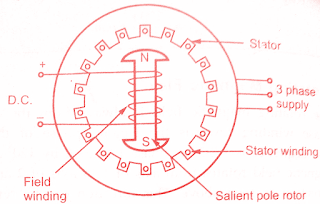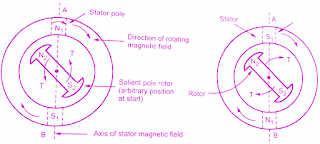## Construction & Working principle of Synchronous Moto:

We have seen the various features of three-phase alternator.Similar to a d.c generator it is a reversible machine.If a three phase supply is given to the stator of a three-phase alternator, it can work as a motor. As it is driven at synchronous speed, it is called Synchronous generator.So if alternator is run as a motor, it will rotate at a synchronous speed. Such a device which converts an electrical energy into a mechanical energy running at synchronous speed is called synchronous motor.The synchronous motor works only at synchronous speed and cannot work at a speed other than the synchronous speed. Its speed is constant irrespective of load, no doubt, its speed changes for an instant at the time of loading.
In this chapter, we will discuss how synchronous motor works and working principle of three-phase synchronous motor.Before beginning the study of the synchronous motor, it is necessary to study the production of the magnetic field. The rotating magnetic field (RMF) is very important to understand the working of a synchronous motor.

### Construction of Three Phase Synchronous Motor:

Similar to d.c machine where there is no constructional difference between a generator and motor, there is no difference between the construction of synchronous motor and the alternator, both being the synchronous machines.

The synchronous motor construction is basically similar to rotating field type alternator. It consists of two parts :

i) Stator: Consisting of a three phase star or delta connected winding. This is excited by a three phase a.c. supply.

ii) Rotor: Rotor is a field winding, the construction of which can be salient (projected pole) or non-salient (cylindrical) type.Practically most of the synchronous motors use salient i.e. projected pole type construction.The field winding is excited by a separate d.c supply through slip rings.Construction of 3-phase synchronous motor

Must Read:

### Working Principle of Synchronous motor :

Synchronous motor works on the principle of the magnetic locking. When two, unlike poles, are brought near each other, if the magnets are strong, there exists a tremendous force of attraction between those two poles. In such condition, the two magnets are said to be magnetically locked. If now one of the two magnets is rotated, the other also rotates in the same direction with the same speed due to the force of attraction i.e. due to magnetic locking condition. The principle is shown schematically in the below figure.Principle of magnetic locking

So to have the magnetic locking condition, there must exist two unlike poles and magnetic axes of two must be brought very close to each other. Let us see the application of this principle of synchronous motor.

Consider a three-phase synchronous motor, whose stator is wound for 2 poles. The two magnetic fields are produced in the synchronous motor by exciting both the windings stator and rotor with three-phase a.c. supply and d.c. supply respectively. When the three-phase winding is excited by a three-phase a.c. supply then the flux produced by the three-phase winding is always of rotating type. Such a magnetic flux rotates in space at a speed called synchronous speed. This magnetic is called a rotating magnetic field.

The rotating magnetic field creates an effect similar to the physical rotation of magnets in space with synchronous speed. So stator of the synchronous motor produces one magnet which is as good as rotating in space with the synchronous speed. The synchronous speed of a stator rotating magnetic field depends on supply frequency and the number of poles for which stator winding is wound. If the frequency of the a.c supply is f Hz and stator is wound for P number of poles, then the speed of the rotating magnetic field is synchronous given by,

Ns = 120f/p   r.p.m

In this case, as the stator is wound for say 2 poles, with 50 Hz supply, the speed of the rotating magnetic field will be 3000 r.p.m.This effect is similar to the physical rotation of two poles with a speed of Ns r.p.m.For simplicity of understanding let us assume that the stator poles are N1 and S1 which are rotating at a speed of Ns.

The direction of rotation of the rotating magnetic field says clockwise. When the field winding on the rotor is excited by a d.c supply, it also produces two poles, assuming rotor construction to be two poles, salient type. Let these poles be N2 and S2.

Now one magnet is rotating at Ns having poles N1 and S1 while at start rotor is stationary i.e. second magnet is stationary having poles N2 and S2. If somehow the unlike poles N1 and S2 or S1 and N2 are brought near each other, the magnetic locking may get established between stator and rotor poles.

As stator poles are rotating, due to magnetic rotor will also rotate in the same direction as that of stator poles i.e. in the direction of rotating magnetic field, with the same speed i.e. Ns.

Hence synchronous motor rotates at one and only one speed i.e. synchronous speed. But this all depends on the existence of magnetic locking between stator and rotor poles. Practically it is not possible for stator poles to pull the rotor poles from their stationary position into magnetic locking condition. Hence synchronous motors are not self-starting. Let us see the reason behind this in detail.

### Why Synchronous Motor is not self-starting?

Consider the rotating magnetic field is equivalent to the physical rotation of two stator poles N1 and S1

Consider an instant when two poles are at such a position where the stator magnetic axis is vertical, along A-B as shown in the below figure(a). At this instant, rotor poles are arbitrarily positioned as shown in the below figure.

At this instant, the rotor is stationary and unlike poles will try to attract each other. Due to this rotor will be subjected to an instantaneous torque in the anti-clockwise direction as in figure(a).(a) Action of Synchronous Motor                     (b) Action of Synchronous Motor

Now stator poles are rotating very fast i.e. at a speed Ns r.p.m. Due to inertia, before rotor hardly rotates in the direction of anticlockwise torque, to which it is subjected, the stator poles change their positions. Consider an instant half a period latter where stator poles are exactly reversed but due to inertia rotor is unable to rotate from its initial position. This is shown in figure(b).

At this instant, due to the unlike poles trying to attract each other, the rotor will be subjected to torque in the clockwise direction. This will tend to rotate the rotor in the direction rotating magnetic field. But before this happens, stator poles again change their positions reversing the direction of the torque exerted on the rotor.

Key Point: As a result, the average torque, exerted on the rotor is zero. And hence the synchronous motor is not self-starting.

Note: The question is obvious that what will happen if by chance the rotor position is in such a way that the unlike rotor and stator poles are facing each other? But owing to the large inertia of the rotor, the rotor fails to rotate along with the stator poles. Hence again the difference of position of magnetic axes gets created and rotor gets subjected to reversing torque.

This is because the speed with which rotating magnetic field is rotating is so high that it is unable to rotate the rotor from its initial position, due to the inertia of the rotor. So under any case, whatever may be the starting position of the rotor, the synchronous motor is not self-starting.

### Procedure to start a Synchronous Motor:

Now suppose the rotor is rotated by some external means at a speed almost equal to synchronous speed. And then the rotor is excited to produce its poles. At a certain instant now, the stator and rotor, unlike poles, will face each other such that their magnetic axes near each other. Then the force of attraction between the two pulls both of them into magnetic locking condition.

Once magnetic locking is established, the rotor and stator poles continue to occupy the same relative positions. Due to this, the rotor continuously experiences a unidirectional torque in the direction of the rotating magnetic field. Hence rotor rotates at synchronous speed said to be in synchronism with the rotating Magnetic field

The external device used to rotate rotor near synchronous speed can be removed once synchronism is established. The or then continues its rotation at Ns due to magnetic locking. This is the reason why synchronous motor runs only at synchronous speed and does not rotate at any speed other than the synchronous. This operation is shown in the below figures (a) and (b).Unidirectional torque experienced by rotor

It is necessary to keep field winding i.e. rotor excited from d.c supply to maintain the magnetic locking, as long as the motor is operating.

Must Read:
So a general procedure to start a synchronous motor can be stated as:
1. Give a three-phase a.c. supply to a three-phase winding.This will produce rotating magnetic field rotating at synchronous speed Ns r.p.m.

2. Then drive the rotor by some external means like diesel engine in the direction of the rotating magnetic field, at a speed very near or equal to synchronous speed.

3. Switch on the d.c supply given to the rotor which will produce rotor poles. Now there are two fields one is rotating magnetic field produced by stator while the other is produced by the rotor which is physically rotated almost at the same speed as that of rotating magnetic field

4. At a particular instant, both the fields get magnetically locked.The stator field pulls rotor field into synchronism.Then the external device used to rotate rotor can be removed.But rotor will continue to rotate at the same speed as that of rotating magnetic field i.e. Ns due to magnetic locking.

Key Point: So the essence of the discussion is that to start the synchronous motor, it needs some device rotate the rotor at a speed very near or equal to the synchronous speed.

#### Conclusion:

Now here we have discussed construction and working principle of synchronous motor.You can download this article as pdf, ppt.

Comment below for any Queries.